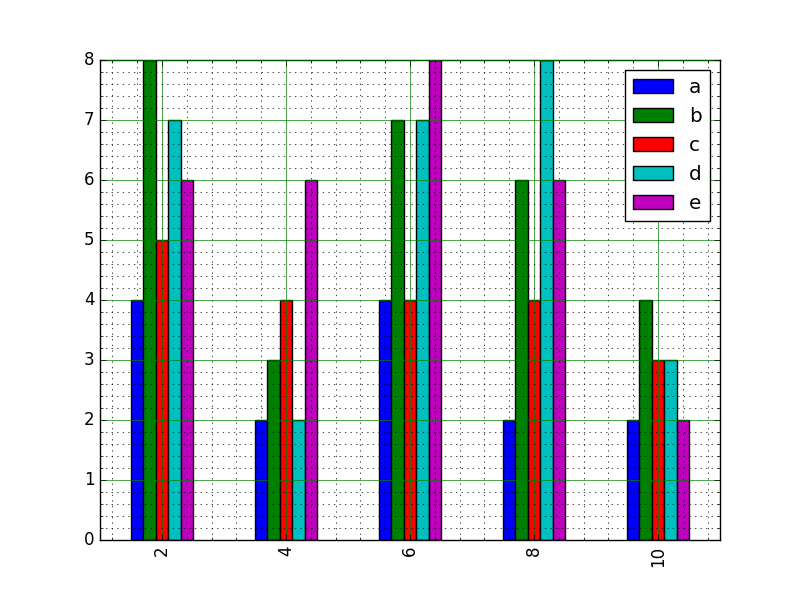﻿ Matplotlib Bar Chart: Create bar plot from a DataFrame - w3resource# Matplotlib Bar Chart: Create bar plot from a DataFrame

## Matplotlib Bar Chart: Exercise-11 with Solution

Write a Python program to create bar plot from a DataFrame.

Sample Data Frame:
a b c d e
2 4,8,5,7,6
4 2,3,4,2,6
6 4,7,4,7,8
8 2,6,4,8,6
10 2,4,3,3,2

Sample Solution:

Python Code:

``````from pandas import DataFrame
import matplotlib.pyplot as plt
import numpy as np

a=np.array([[4,8,5,7,6],[2,3,4,2,6],[4,7,4,7,8],[2,6,4,8,6],[2,4,3,3,2]])
df=DataFrame(a, columns=['a','b','c','d','e'], index=[2,4,6,8,10])

df.plot(kind='bar')
# Turn on the grid
plt.minorticks_on()
plt.grid(which='major', linestyle='-', linewidth='0.5', color='green')
plt.grid(which='minor', linestyle=':', linewidth='0.5', color='black')

plt.show()
```
```

Sample Output:Python Code Editor: